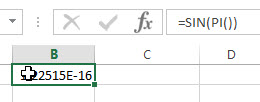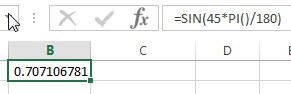# Excel SIN Function

This post will guide you how to use Excel SIN function with syntax and examples in Microsoft excel.

### Description

The Excel SIN function returns the sine of a given angle. If you want to supply an angle to SIN function in degrees, then you need to multiply the angle by PI()/180. Or you can use the RADIANS function to the angle to radians.

The SIN function is a build-in function in Microsoft Excel and it is categorized as a Math and Trigonometry Function.

The SIN function is available in Excel 2016, Excel 2013, Excel 2010, Excel 2007, Excel 2003, Excel XP, Excel 2000, Excel 2011 for Mac.

### Syntax

The syntax of the SIN function is as below:

`=SIN (number)`

Where the SIN function argument is:

• number –This is a required argument. A number that used to be calculate the sine of an angle.

Note:

• If your argument is in degrees, multiply it by PI()/180 or use the RADIANS function to convert it to radians.

### Excel SIN Function Examples

The below examples will show you how to use Excel SIN Function to return the sine of an angle.

1# get the sine of pi radians, enter the following formula in Cell B1.

`=SIN(PI())`2# get the sine of pi/2 radians, enter the following formula in Cell B2.

`=SIN(PI()/2)`3# get the sine of 45 degrees, enter the following formula in Cell B3.

`=SIN(45*PI()/180)`Or using the another formula to get the same result as follow:

`=SIN(RADIANS(45))`### Related Functions

• Excel COS function
The Excel COS function returns the cosine of a given angle. The syntax of the COS function is as below:= COS(number)…
• Excel ACOS function
The Excel ACOS function returns the arccosine value of a number.The ACOS function is a build-in function in Microsoft Excel and it is categorized as a Math and Trigonometry Function.The syntax of the ACOS function is as below:= ACOS(number)…
• Excel ACOSH function
The Excel ACOSH function returns the inverse hyperbolic cosine of a number.The syntax of the ACOSH function is as below:= ACOSH (number)…
• Excel ASIN function
The Excel ASIN function returns the arcsine value of a number.The syntax of the ASIN function is as below:= ASIN (number)…
• Excel PI function
The Excel PI function returns the value of mathematical constant PI. The returned value is 3.14159265358979 and ti will accurate to 15 digits.The syntax of the PI function is as below:=PI()…
• Excel TAN function
The Excel TAN function returns the tangent of a given angle. So you can use this function to calculate the tangent of an angle in radians. The syntax of the TAN function is as below:=TAN (number)…